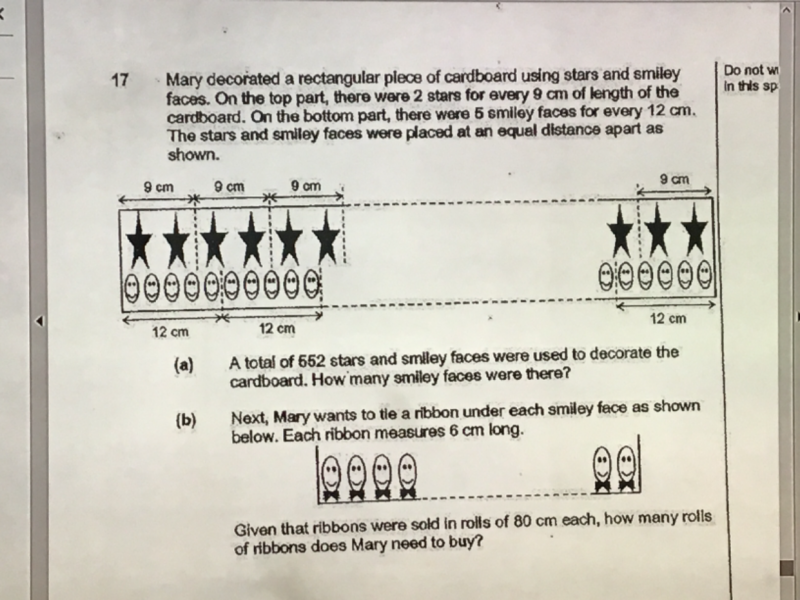# QuestionPls help to solve (a). Is the answer 360 or 864?

Hi Aaron,

A total of 552 stars and smiley faces were used so the number of smiley faces MUST BE LESS THAN 552.   So it can only be 360.    864 > 552.

However, it can be calculated as well.

For the stars and smiley faces to meet exactly,  4 x 9 = 36 and 12 x 3 = 36

This means 4 sets of  2 stars = 8 stars and 3 sets of 5 smiley faces = 15

8+15 = 23

552 / 23 = 24 sets of 8 stars and 15 smiley faces

So total smiley faces = 24 x 15 = 360